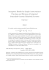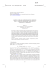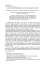# Document 6527798

## Transcription

Document 6527798
```PKOmBliLITY
AND
MATNaFufiATICAL mAml;[email protected]
Abstract. The rate oi convergence of monotone approximation
gromdures for stockistic promsses i s studiwl, Several applications are
discussed: dismeti7;ing time sefics, constructing solutions of linear
stadastic daerentisial equations and sirndating aistrlbutioas of continuom Ji~nctiantals.
8. XrmtrdrrcGsn Approximation ef stochastic prowses has 'been studied
under. various aspects, e.g. converpnce of empi~calprocesses with cadlag
sample paths Pillhgsfey , Gsnssier and Stute , Pollard [l6]), simnlating fiaxlcarands af contjlruous Gatussizltl processes (EpTetti [93) and approximation of weakly stationav random processes by convolution processes (SplettijtBsser >. According to \$iffereat objectives, the risults are based on quite
different assump~onsabout the underlying procmses and apprcsximdioa
procedures,
It is: the aim of this pager to debva qumtitative results on monotone
approxhatian of stochaslic processes, i.e. approximation by operators gaeserving some order ioxlaiions, The dass of monatunc methods in~ludash e a r
interpolation, qprofoxirnation by step Fundilons, interpaEdtian with momalized
B-splints and approximatian by linear integral operators with nonnegative
k~rnels.Approximatioo by a sequence TI,
I", , .. of monotone operators: is
stcirmtageoirs bacc~usethe asymptotic behaviour af the approxima~anerror i s
Iu&Q.
For the moment kt f ( t ) be a csntinuoua Fancrtim defiled on a closed
interval [a, b] c R. Then the following properties are welt-known:
(1) If* for n 4 caplirnfa",f')l(r) =J(s) (in the sup-norm) has been proved for
the ""grasy" fufunsiians .f,(t] = 1, (r') = t, f 2 (j) t2, then ?nlirn (T,J)(r) = f ( t ) i s
-
rn + m
dready tme for each SO)E C [a, b3.
(21 Tbe rate of convergence far arbitray J(t) E C [a, rjl can be specified
provided the rate of csnvergensf: is known for -fa (0, f i (tj and f, [f).
Hence it suf5ces to check three ""test fufutlctioas'banly. (These is an extensii~e
literature on monotone approxima~anof nonrandam functions, and there nee
many extensions \$0 more general fmction space\$ than C[a, Erl. Important
results are given by Anastassiou [I], Bereas and Lorentz , Censor [613
B V o r e , Donner 181, klah , Mond and Vasudevan Cts], Woth [17j2
scheafold [I 81, Sclzempp , WoIE f229. Clearly, this enr~meratilonis by no
means complete.) Under mild additional assumptions property \$1) has been
establiskd for stackastic processes by the author [213 while pneralizationra of
property (23 are treated in this paper. Several applications are discussed:
discretin'ag stationary time sea-ies with trend, constructing solutions of linear
stochastic differentid equations and simulating dist~butionsof certain fasnctionals of nan-Gaussian processes. The last problem origilzates from Eplett [3).
2. Basic defimitism. Throughout the text X t t , a) stands for a stochastic
process on a probability space (a,d , P) with the dosed interval
[a, b] c R, a < b, as pmmieter set and state space ( R , B')3where \$3 denotes
the (r-field of Bore1 subsets of R,
Sums, pr~dttctaetc. of stoctdastic processes are defined pointwise and the
natural odering X sg Y iffX(t, 4g Y ( t , w ) for each t ~ [ a\$1
, is used, (It will
not be distinguish4 bemeen equivalent prclcasses which differ ody with
grcrbabif ilty mro for each I E [a, b]J
The vector lattice of &all processes with bomded second m m ~ n r i s
is defined to be
[]XItpw)ll a = ( E IX (t,
and becomes a narmed vector lattice by means of the aerm
lixll
-
sup Il%lh aSllz.
setn,bl
Fhe space of Lz-contjauzrus procesms, Ca la, bl = C ( [ a , b ] , L2 (a,sf, P))l
whew la, 6;a is endowed with the aaturd metric, i s a linear subiattice ofla, [a, b].
The sflaws B &a,b] and G [ a , b] of sserandurn bounded a d nenradom
eontinunrrs rcal-valued functions may be ~mbeddedby identifying a nonstrschast j function
~
f ( t ) with the degenerate prsfocws Xf(t, W ) =f(21, t E [a, b], m~ i2;a.
Note that for every X E Bra[a, trl ( X E C, [a, b ] ) the anean value fwcehon
(EXj ( t )= E (A7 (t, w)) and the "'noj-rn function" XI,( 1 ) ItX ( 1 , C I I ) / ~ , namssarily
lie in B [a, b] (C [a, b], resp.). Finally, smac3thiless of a process X e 8, [a, b] i s
expmssed in terms of its stochastic modulus of continuity:
-
3. Moaotom ~ ~ f a t 8from
m %[a, h] into B,[a, b]. We ibegh with
D c f i n il i o n 3.1. A baunded linem mapping T: C, [a, b] -+ B, [a, b] is
called monotone if X 2 0 implies T X >, 0 for each X E CD[a, bf,
On the c ~ n t r a qto the nonrandom case two additional conditions are
squired in order to obtain stochastic analogues of propmrfies ( 3 ) and (2) which
have been considered in the intraduction. Hotvever, these restrictions arc
satisfied by every monotone operator of praeticd importazzce.
The first corvdilicrn sirnply states that taking expectation is interchangeable
with application of T.
Definition 3.2. A mapping T : C,[a, b] -+&[a, 63 is called E-cornmtattatige if E ( T X ) ?"(&X) is true for each X E C, [a, bj.
The secand condition may be interpreted as folljows: the image of
a "simple" pmrocess X,, may be v i e w d as a deformation of X I A ,For each event
A E & the "simple'>p~oeess XI, E C , [a, b] is defined to be
-
Definition 3.3. A mapping T : C,[a, b] +B,[a, b] is named s t ~ c h s tically sinzgke If there exists a zeal-valued function a,: [a, b] -+ R such that
( T X i A(t'
) W ) = aT(t). 1 A (UF), for each t E [aCa,
b], is valid for every A E ,d.
The operators mex~tianedin the introduction are automatically E-cornmutative and stochastically simple; see ~ B Weba
O
[ZI], p, 75.
LEMMA
3-4.[i) If T is E - G O I ~ Z P then
~ Z ~TCmaps
~ ~ ~the
~ Usubspace
~~
C KG, b] infa
BCa, bj.
(ii) t e r T be monotofie, E-commutative .laud stochascicaliy simpk. Then, far
cmk " fC~[a, b] and ~ a c hrairldsm variable Z (w) with 11Z (a>
11a < r;o, the image of
the process f'(r ,60) =f ( t ) Z (a)satisfies (7jf') (t , wj (TJ)(2) - Z f w),
Proof. fir l"f= T(EJr)- E [ T f ) lies in BCa, b] for every f ~ C : [ i ab].
~
(i.i)l Starting with an indicautor variable 2 (w) .= 1, (w), A ~ dthe,imquality
-
-
-lf186~A14
6 f ( 9 - I n ( U )d) IlfII [email protected]^) implies
-[If il +.,[email protected]" l;J(uT)G (Tfi,l(c. 4 4 III'll
-
'a,(t).
[email protected]
Hence (T'& (t "u) 0 if OI # A. Repeating the same argumnt with 9 -A
instead of A we crbtain {TI
,)(t, m) = (g)
( t ) . S A [w) bsause of
If Z ( a ) is an arbitrary v~riable,wc may r;fionsr: a sequence Z,,(LU)
of
prhitive vstd.iab3es with lim I1Z ( r ~W
) Z(
, ~a )= 0. Tlms
meal
~~~ 1I 12 -f2,r11 = 0
lI"ff2
is true and the crsnril~uityof T yields (TfL)[t,
w) (g)(o.Z(ur).
The next lemma is dealing with a sloehastie vewiiun of the irrequaltity
fg[
d TjfE whifnjich holds true for every f~C [a, h], For monotone hornamor=I
phisms an vwtor lattices this inequdity m;ky be verified immediately, Hawever,
the stochastic analogue i s not wi&nt since the A,-norm I).X(x, m)[{,is rlikrent
from the absolute vdue IX(2, @f,
LEMMA3 5 ;Let T: C, [a, bJ + B, [a, b3 be monotone, E-cowmutative and
simple, Then (TX),!d T(XII) .fix+each X E CLa[ a , bJ,
P r oaf. Hold O ELa, b] fixed.
(i)Define a mapping p,: C [ u , b3 -+ R by r;o,Cf*) = (g)(t).
-;y, is a bounded Zillear func~o~zat.
an C [ a , bl; therefore, the &esz
representation theorem guarantees the existence of iifunction q,: [a, bj -+ R of
bounded variation such that rp, can be expressed as a Stieltjes integral
By manotonicity, cl, can be assumed to be asndecrmixlg.
(ii) The nzapgngs @\$, !Pt: C,[a, b] -+1;,(52, d , P), being defjned by
aad fY,(X) = ( T X ) ( t , a),are bounded line= operators extending rp,. Since 4, is
aondecreasitpg, we gee
Furthermore, if Z(mJ is a random vakblle with \/Z(co)ti,< m and
f~ 12'pa, b], then the processfZ(s, cu) --f (\$1- Zfro) [email protected], (fd.= fjbff)'2[to)
and ?u, (fi)= "ul.(f)- 2 (a)
(see lemma 3-4(\$1. Consequei~tly,Br, and "P, coincide:
on the subset Da [a, b]* where
@g
-
But D, [a, b] is derrse in C, [ a , bl
( X ) Yi (X) for eaeh X E Gi2[a, b].
(see, e.g,
BaurfsaE &S]).
Thus
(iii)Finally, we obtain
C ~ R ~ L L 3.6,
A RThe
Y aDvnr I/ TI1 of a maaotorze, E-comutatiw arid stackstielzliy simple operator J" .~ati~\$es
11 Tll = lws[l.
Proof. For eaeh X E C,,&, 63, Xll(t) = IjXiZ, tll)[lz % IIXII.
113
Monotone appraximrion
Since T maps the eonstant function [IXI/ on ][XI3 a , (t), lemma 3.5 gives
4. 'f"hs rate of convergeace. Bounds for the rate of convergence wiil stern
from theorem 4.1 which generalizes m~ultsfor monotone approximation of
detcrmbiskic functions to the stochastic context. In the sequel the functions
fO3fl fzE C [aib] are defind ta be f0 lt) .= 1, f, ( E ) = t, f2 (t)= tZ and for each
fixed C, E [a, b] the symbol h, stands for the function
THEOREM
4-1. ASSMMEthag T: CQ[a, b] -+ BQ [a, b] 13 a M Q ~ O ~ Q E ~ ?
E-comnrsutative and stochastica fly simple operaticvr, Then,far eaeh X E C, [a, b]
and each 6 > 0, o w can conclude that
Proof. Hold t , ~ [ a ,b] fixed, If t ~ [ ab], i s given with It--tQ/r 5, we have
Therefore, the procas Y;,( t , a)= X (t, IU)-X (tQ,m),fO(z) satisfies I:,),,si
h,). Since IF is monetaxle, we obtain T((Y;,),,) & q ( X ; 8) x
q (X; 6) (jb -ix CV0+6-'- Th,,).
Ey lemma 3-5,(T&o)Il
6 q(X; G).(go+IG-Z.Thtp).
The specid case t .= e,
and the relation [TY;,)(t, us)-, (TX)(t, o)--X (to, su)*{vo)(4
(lemma 3.4) yield
*
S h a to was; arbitrary, the ~up-mrmmay be taken an both sides. Thus
?!(x;8)' SUP ((TO)C~)+~-'~(T~~(E))
IIax-x* %It
rrfa.B]
d
6)-{iilTf,ll +d-2. sup f(rh,)(lc)l).
rsla,63
v,
+
'
I
&
-f, E B [a, b] implies ItX* -XI G llX[] 11 'I&
-faj(.
and the assertion
i s a eonsequence of I]TX -.XI[ g I1X. 7;- X ] fiTX -X
fl.
C o ~ e ~ ~ 4.2.
a a vCottsr'der a squencu "I ;:f monotone, E-commacfative and
sioelzastkally simpi!e opRators. If 6 , is a seqagaee 01positiw seai numbers
satigying sup
h,)(t)!G ylS: fa7 solr-se cnnst~a~rry 3 0, than
rHaA
In pmticular, the convergeme Em IlX - x.X 11 = 0, n -, 00) can be msamed
for each X E CQ[a, B1 provided lim 11f- T,f 11 == 0,PZ -+ m, laas been vw(fiedjbr
each j r 4 Y O * f I * f f 2 ) *
Co~oUary 4.2 may be used as follows. First, it must be decked if
wsonvergenGe occurs for the three '"test functions" fo ,fx,
j', . Then the rate of
convergence dbr XECC,
[a, b] is detemined by the expressions sup I(T,hJ ft.)[,
t e [ a , b], and the smoothness of X beiug measul-ed in terns of its stschastic
modulus of continuity. For vadous sequexleEs of monotone operators, bounds
for sup l[T,h,)(t)[,8 E [a, b], cm be found, e.g., in DeYore ; typical squeaca:~
are Jb,= a-' or 8, = n-li2. (The operators considerd in the literature are
defined an C [a, b] rather than oa 6 , [a, b] and usually admit aepresentations
.with fixed points t , ~ L a ,bj and presc~bedfunctions a,, K,,K , . A process
X E Csa[a, b] is then approximated by T X on the tacit understanding that we
m dealing with the obvious extensjam
k
(TX)(f, fol =
k=l
B, @)- XCt,,
ts
03)
or ( T X )(2, o)-- [K l (s, t) X(X2(s, if), m)ds,
a
where the intkgrd is to be inteqreted as stmhas~cRiemanu integal in the
L2-sense. Note: that both rep~sentationsguarantee Ec~mmutativiQ and
stochastic simplici~.)
It will always be m~ssumedia the sequel that 9", is; a sequence of ogeaators
satisfying the conditions of carallaxy 4.2 with l h 6 , -- 0, n 4 m. For 'canvenienee, 'F,fO . f , is a l s ~assumed which holds true for many moncntom
operators. Settiflg f l - 1i - 7 we get /IX- X ([ B 8.4 [ X ; an) and
lirn q ( X ; 6,) 0, rz + w, for each X E C, [ a , bJ, thee t h i quantiti~sfl and 8,
have: been determined, wc must cxlnsider q{X;&r), w&ch depsads only an the
autoccrvadmce strtxeture af the process utlae~con~ideralbion,
In the lolllowing sectinns three app~cationswe dbcassed; other problems
may l
x treated similarly.
-
5 Dkredza~on0%s3a6ionary time series d t h t m d . Let X be a time series
in continuous time. Far comput;ttional purposes, some digeretized version d
X must always be used arid the quas~onarises whether it is possible to specify
bounds for the discl-etization error in order to controI the inevitable bsw of
infarmation.
A standard madd for W is the faUowiagt X allows rr dacampositian into
sr nonrato~hatic trend component f and a weakly statisnary stochastic
cornpolrent with mean value function (Et)(t) = O, a e t G B, and auto~ovariance:~ X I C ~ O I PKt(z), -(b -a) % s < (b -a). Smoothness of K,: at the
origin tviU be expressed in kerns of the Local modulus of conGnuity
11s
Monrrtclrrs approximation'
q*(K,;S)=sup1K5(~)-Kc(rr3;F=K,(0)-id aEKgi(.e), O d 6 f 6-a,
OItid
ItlCb
and diflerentiability of t i s to be rrndmitood h the L2-sense. Furthem~re,the
uninteresting case 6, > b-a may be exduded,
THEOREM
5. C Q I Z S ~
the
~~
time
S Tseries X (E,o)=.f ( t )+t jr; m), t E [a, b], where
the nonstachasliic trend \$ is an ebmmt of G [a, b2 and the weakly statitnsry
process Q lies in C,ra, b], Then
with a, = pB'a2= P-.J!%
/IX-%XI1 6 a , - q ( f ; 6,)1a2.J13..=
k;ff a d are diferentiabb wieh f" E C [a, b] nnd E C , [a, b] ople obtains
IlX-TX'll < ~exS,, w h r e ril= p(!lf l \ l +
Proof. The first inequality is a eoassaueloce of the relations
sW; 6 ) 6 r l ( f ; 6 ) + q ( C ; S )
q(t; 6 ) ~
The diRrerentiab3iQ conditiom \$eld q(9*; 83 g 11f'li 8, a d ensure that the
second derivative of Kt exists and is cantinuhus on [-(b -a), (b-a)]. The
semnd inequality follows by Taylor's theorem and K; = 0.
6. SoJ~iaglinear stacbslstl: M e r s ~ ~ s lquaklaaas*
l
Comider the formal
stochastic differential quation
e;Xh~(lt, a)+... + ~ ~ ' X l m
( t) -,t - e , = X [ t , w ) = Y'@,.w)
with initial eonditiom X (a,*m)= %' (a, a)= ... = XIM-lk(a, w) = 4 given seal
numbers c, (nz & 1,c, tlC: et) and a fixed rime point a.
The deri.vative T' (t., w ) of the prowss Y(r, @ may not exist. Therefore,
a pracess X (t, ar3)is caged zr. solu~oraof the equation if X (t, w ) satisfies the
initial conditions as well as the integrated equation (in the LZ-seme):
1
cm [email protected]"'l~(f,
.
@+
... =+c,.X(i.,LO)'~"C*.JX(S,
Yft, 1~)--3T(i2, CO;).
SL))~S==
rt
Many phenomena in
pbysilps and enpeering sciences can be
desefibed by this equation and the problem occur5 to compute the solution
PI-ovidedsome,id'amatjc)nabout. the for~iragfunction B i s avagabls, TypicaHy,
an approximation 4", Y af E" must be used where 9", I.'is based on observations
from Y nt sertain time points. h dated problem is the fcrllot;ving: it is possible
to vary the wefieient s c, , .,.,c, and far each set of c~e&~ients
the sample
path belzaviour i~>fthe solution has to be studied. tn that case sampled versions
of Y wodd he gnerated by simtlhtim. If the forcing function Yfr, a) has
oxrhogamal inerments, a solution of tke dserential equation can be comtracted on each interval [a, b].
The symbol cL, will stmd .FOE
.
THE~RE
6.M
A~sszimY(t, 0.1)is an %;k-eontirluoeeyocess with meail U ( I ~ U &zero
and lrrrthogo~mlincrements a ~ suppose
d
(TIY)(t*cow)E C, [ta, It] for each rz. Lst
X (r, o),t E [a, m),be the unique salbttiorl to the above initial m l ~ epuobFer~and
EEC 0 be Gree~r'sfunction of the nssfsociated ma~zj.arzdanzhomo~eraeoxrsinitial whe
probimt,
Q) Set
(s,x)ft, mE = ~ n m ' I xy)(tl m ) - g ( f - n f - ( m F)(rr, a)+
f
-t- [email protected]'(s-s)'(T;,Y)(s,
wlds,
I:€[&
E7.J.
a
Tlem [IXI-S,XII 6 cl-q(P; 6,) with
b-a
a=PqOc,,,t+
lu(s1l-t ,f fg'(4ldgS-
sup
BSsCb-a
0
(ii) I ' m 3 2 ~ V r dY(w, w) is Icrz~wn,set
( ~ : x ) ( t , ~ ) = - g ( r - a ) - ~ ( n , o ) + i g ' ( f - s ) - ( ~ ~ ) ( s ,ttCo,bl.
~)ds,
a
Then
b-a
P r a o f. The solution X {t, u),t E [a, a),is given Isy
r
x(k,a):
= fg(t-s)dV[s,
m)
U
(see, e.g., Ash and G a m e r C23). Sntegatian by parts yields
1
X ( e , Lb)) = g(O).Y{t, ru)-g[t-iaj.
Y(n, ro)+ I g ' ( r - - ~ ) -Y{s, O ) ~ S W
The assation fallows from g (0)= c,
and
A s ~ l a ranlt
r
may be derived for arbitmry initial conditions, '
Provided (IT, Y);ttS(s, w) depends on a finite number of observations from
Y(s, co] - the only case rzf practical relevance - (qf , ( s , cci) admits a representation
Kfn)
@). Thus (5,:x) ( t , lo) becomes
with tk\$,,
E [a, 51 and nonnegative functions
a Tinear cambhatioa sf the observations,
with coefficients
I
dk,fiC1) z=
elm'BA,rt(t)-~
jsF(di.-s)'Dk,nCs)ds=
It-a3.Dk.o(lil)+
Q
If rn m 3 and the left endpoint a is an observation poiat, say a
obtain
CE x3 (4 4 =
-
t,*,,, we
z
dk",nlo. YCtk,ylr 4
k- 1
where
I
df,lt[t) = - ~ I t - a l - b f ~ ' ( t - ~ 1 ' D 1 , , , 1 ~ ) d ~
0
2
and
d2, (t] = 1g' [t - 5 ) .
( 5 ) ds7
kc 3 2.
il
In many instznces Green% function g can be wlculated easily; the
UhIenbeck-Omstein, process as a salution af a second-asder digerential
equation [email protected] serve as an example,
T:be most important special case arises if the for~iagfunction Y is
a Brownian motion with variance rr2. Then the moddus of contjnuity fvUils
ox.
rl(Y;83=
The appraxhation S, X in theorem 6 sterns from an irllegral representation
of the solution X in terns of the forcing function. Since the integral is
a ~ontinuoustrm~formationof llrc integrand, any integral relation between
solutian and forcing function yidds an approximation S, X with
lim HX--S,XII = 0,n -+ m. For instance consider a linear stochastic differential equation where the coeffieieats ci = ci(t) are allowed to d ~ p n d
on time t. If
the roots of tile characteristic equation are bound& away from the imaginary
axis, an integral sepresevleation may be obtained by application of the partial
fmction e-xpansiotr method (Kaopmans [I 43, chap. 4).
7. %muratingEEistrjbntion~af fu11cFiomaIS.In order to salve ereain problems
dealing with ampit-icd pmcesses: or tes~nprof hypotheses one has Pa know the
distribu~onof qo OX,where y.l is a fuirnctiand rigdined on a s~?tof stoclnastia:
PE the &st~butionof rp e X Galanot be determined directly, one bas to fall
back upan simul;ictions. Fss obtaining the: ayanptotic distributions of rank test
statistics, Eplett [10, 113 used apgrexirnations
of X to simulate the
distribution of rp s X by simulating qr, o 2,, En
Eplett gives a general treafis~
x*
of this methad. Quan.titative results \$or q~o x , -+ tp io X (convergence isn
distribution). are dierived uncle; the following assumptions: X is a Carasslan
process on LO, 13 with mean value ~ r and
o
P {X(r , o)t
z C [0, 19) 1,
p: CLOTItl-*R is a esnhuorns fun~tional, m d \$, i s Ibe mbimoem
L2-approximation with respect to a prescribed subspace of Lm[0, I]. The
Gaussian property and continuity of the sample paths me swt6ctive, and
EpEett p s e s the proMem to charaderize convergence for non-Gaussim
processes
p. 181). If T,X is substituted for
c e r t ~ nfunc~onalsIs,
Usage of G X has advpntages: X is allomd to begong to the large class
C,[a> b], no smalytied proprties sf the s;nmpte.paths are rqqtuired and no
assunrptions have to be made about the distsibutiem of X . This may be.
helpful; for instsaw, if cp o X is interpretd as test statistic, the Gawsian
proprty of X is often satisfied merely asymptotidly, Another eiample is the
PolloGng: X is a process with caaag sample paths which have jump
discontinuities. Udortunately, there is also a drawback. Due to the norm
4=
functknds such its q~o X = sup [ X ( t , 41, t E [a, b], cannot be treated. However, it is possible to describe the rate of convergence far impo~ant,subclsisses
d fu'unctionals, e,g, furrctionals related to Lp-noms. As an jfiustra~on,the case'
.
is discusgd where k has a h i t e totd variation [email protected])on [ a , 313. Wewlt that X i s
&'-~nthucrus, hence X2 is Lx-continuous on [a, bf and @,OXis a anre&
dehed wndom wluiable with finite expectatlotn being the L t - l ~ stf Stieltjes;
sums, kt. (q,,
denote the L&vy distanw batween the \$istPibution
functions of ys, o X and 9,s X.
Zk~c~azau
7. Suppose X E CQ[a, b] fir each n, 9"llea
+
liln pp,oT , X = phaX
n-tm
is ucalid
in the L1-sense &d the Lduy distance [email protected] L: (p,, %) 4 i< ?J (X; 8,J9
ct = 2p. Y(l8).SlXil.
~ r b o f .or each XEC,C~, b] one obt.ins
whre
a.
- -
Sinw jo = qfD
has beell assumd, carallary 3.6 gives ]IT,/] I[aT,,tl I.
Therefore, E jcp,o X -q ,o "jr, XI G a q ( X ; 6,). Hold 8 E R fixed, Then
is [rue for arbitrary E r 0.
By Markov's inequality,
r~(X; \$3= 0 implies Ln(p,, X ) = Q and if a q (X; 6,) is positive, the
. This completes the proof.
assertion folows by setting E =
Again, consider the special case
a
with nrlnnegati~efuctisns D,,,(t) and obs~mationpoints
c,,,E
[a, b]. Then
is bilinear in the observations vvith eotEcienrs
El ] G. A. An as t a s s i u,~ M~SGCJERP~~~UUS
sharp rrrequalities ard Koronkirr-type conu~rgcnce
[email protected]@~s
[email protected] aprobability rnemrga, J. Appr~x.Theozy 44 (1915], p. 384 330.
EZ] W. 8,Ash sad M P. Gatdaer, Tapirs En stol-ila&c processes, Academic: Plresa, W m Y ~ t k
1975.
N.B e r m s and G. G, C a ren t F+ Theor~msof Klnnukir~type for positiue liasw opcruatms on
&nwk lattices, [h:JAppsuxikrat~u~r
theory ( ~ d6.
. G. Lr*ran&),p. 1 34 hmdmic Press, New
Vork 1975,
l43' FnBil lin gale y, ebnof3rgerfee of' probability measrrres, Viiey, Pdttw Y ~ r k1368.
[SJ N. B o urblrkj C T m ~ a llapIegyg p a t 2, Addison-Weslw, Reading 1966.
[iYJ k3. Cen c a r, Quanrftatiwarmuftsfcw porosifivc?linear approdrnrrtbs opwntala, J. Approx, Theory
4 (1971k p. M2i450.
174 R. A. UieY ore, Th&appraxim&lon nfcontin~elllsfincticrnsby pmitive linear [email protected]%
Lecture
Notes in Mathematj~s293, Spring, Brlia 1972,
f ll] K. Ill o o ne r, Extensions ofposiaw opmators a d Xoraukin tkearemq ibidem 904,Berlin 1982.
C9] W. J. R. Ep'lett, Apprmi~urlOo#ttleury fbr flze sirnulatian 4 enntinuous Gous.rian processes,
Probab, %. Rel. Fidds 73 (1985), p. f 59-181.
LlO]
The [email protected]\$yof ifnear rmk tests mder i5ahdw [email protected] Ann. Stat. 9 (1981), p.
1979- 1086,
[I 11 - %a Mm-Whitmy: .type rank texts, f. Kay. Stat. Sac. 8, 44 (J9XZ), p. 2'?(3-286.
-
 P. Giinssler and W. S t ut e3 W#hrsch~?~lriirhkeitstI1e~n'e,
springer, 33erlin 1977.
[i3] I,. Rabn, Xlachasfic rmsetlzuuls in conrxeckiun ~Etlaapprosimatiofl ihr?orem for positive Bnem
t ~ p r ~ i oPacific.
~ ~ s , J. Math, POX (19&2), p 3117-319.
L. W. E;s spmans, The spectral anrrljjsis of tirne series, ncadelrti~Press N e w York 1974.
[IS] B. M a r ~ dand R. Vas u d c v ~ z On
~ , upproximatial~by llin~orpesitiae operators, I. Apprhx.
Theory 30 (19801, p. 334-336.
 B. Pal lard, Conuergence aj' stochmtic prac~ss88~
Springerx New 'xlork 11984,
Cf 73 W. Ro t h, Families qf conflex ,nrrbsets md Kor.oakin-~j\$p?pe
tkeorems in bcolly convex spaces,
Prep~at763, Pacttbereich Mathmatik, TH Darmtadt, 1983.
[t&J %. So heSFold, Ein allgenteiner Koro~kirz-Satrfiirbhl-konuexe I'rktoruerb&e, Math. Z. l32
(l973h p. 289-234,
 W. Schempp, A note om Koreokin test families, A d Math. 23 (19.721, p. 521-524.
C203 W, S p l a t t s t G s s er, On the approximtrtiun aj' rondom process^ by c o n u o l ~ i nproccssrrs,
ZAMM 5 (I9&1], p. 235-241.
[2l] M We ba, KuroakiFz system af stmhavtic pwcessq Math. Z. 192 (19861, p, 72-868.
M. Wolf&On the universal Kououkn ebstne ofsubsets in vector lattices, J. Approx. Tbeary 22
(19781, p. 243-253.
[a]
Institub fir Mathemalischc Stohilstik
Uni~emitgtHamburg
Blmdesstr. 55
2 0 0 Elamburg 13, FRO
```

### STOCHASTIC PROCESSES (Prof. W.Woess, Prof P.Baldi) This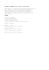### Scalable Control of Positive Systems The Dujiangyan Irrigation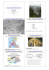### The Essential Spectra of Toeplitz Operators with Symbols in Hâ + C### Fall 2014: MATH-GA: 2111.001 Linear Algebra (one term)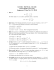### Full text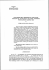### Heat equation in vector bundles with time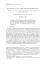### A family of newforms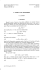### important notice - Mille### SAMPLE COURSE OUTLINE CMTH 500 INTRODUCTION TO STOCHASTIC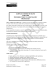### A Uniform Law for Convergence to the Local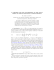### Slideshow/handouts from presentation.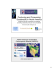### HOW TO GET STARTED AS AN AMRRON OPERATOR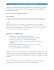### Johnny O`Hara - Plummer Home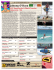### information design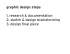### Corporate Factsheet_English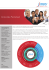### Invariance principles for adaptive self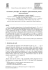### Lower Limits of Type (D) - Departamento de MatemÃ¡tica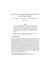### Sample Average Approximation for Stochastic Dominance Constrained Programs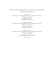### Regularity of the sample paths of a class of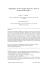### Asymptotic Results for Sample Autocovariance Functions and Extremes of Integrated